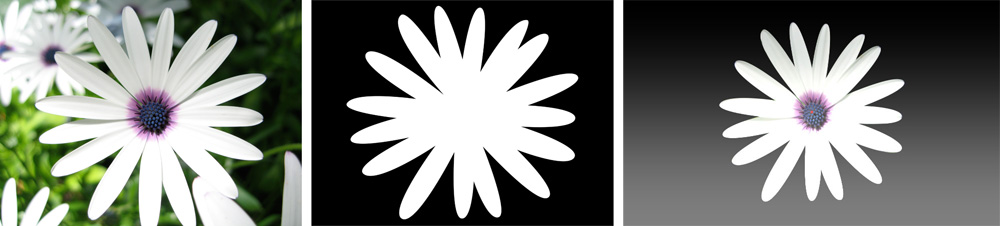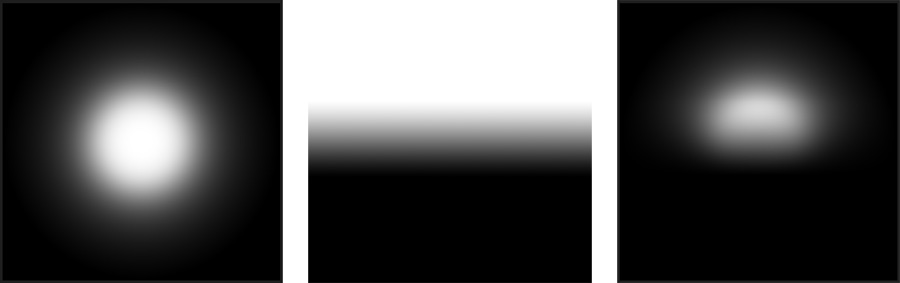﻿ HDR Light Studio User Guide - Alpha Multiply

# Alpha Multiply

The alpha multiply provides an optional secondary alpha that is multiplied by the alpha of the main light content.

Content types for Alpha Multiply are: Image, Gradient, Box Gradient, Scrim Light, Polygon (default)

Alpha Multiply: ImageIf you load an image the value channel of the image is used as a stencil/matte.If you don't have a matte to use in the alpha override, you may find the gradient control sufficient. Linear or radial ramps can be used to cut or shape synthetic lights. Using a gradient can produce a highly controllable soft edge or additional fall off to a light. This is very useful for sophisticated car and product studio lighting. The ramp shape can be controlled and rotated using the sliders.Left: Bulb Content   Middle: Alpha Multiply Gradient   Right: Result